# How to Add Prefix or Suffix into Cell Values in Google Sheets?

Adding prefix or suffix to a set of cell values in Excel is a very-consuming process when we do it manually, however you can complete such a task very easily just by using the simple formula. This article will help you understand how you can add prefix and suffix to cell values.

## How to Add Prefix into Cell Values?

Let see a simple process to add prefix into cell values.

### Step 1:

Let us consider we have an excel sheet where it contains the list of words as shown in below image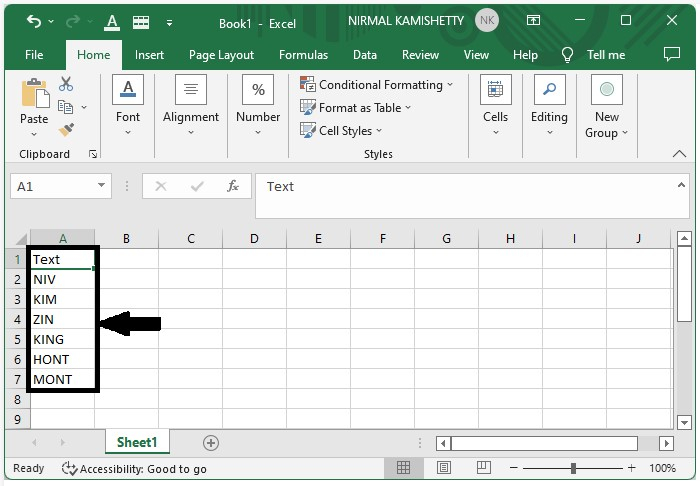To add the prefix, use the formula as "message" & Address of cell

In our case, the formula will be ="NIRMAL-"&A2

Now click the empty cell where you want to get the result and enter the formula as ="NIRMAL-"&A2 and press Enter to get the first result as shown below.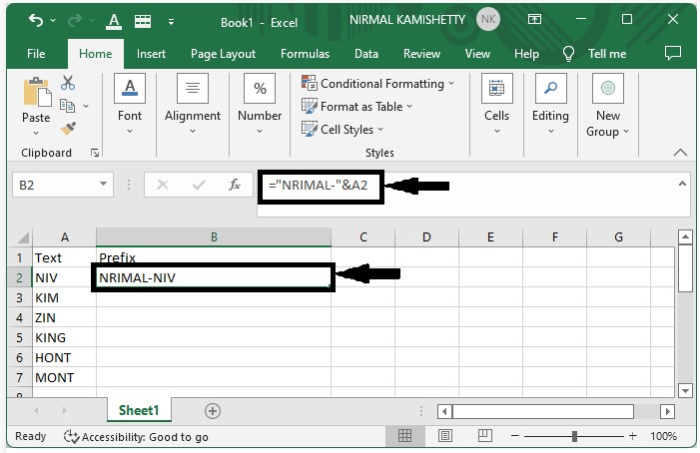### Step 2:

You can display all the other results just by dragging down from the corner of the first result till all the results are filled. The final output will look like the one shown below: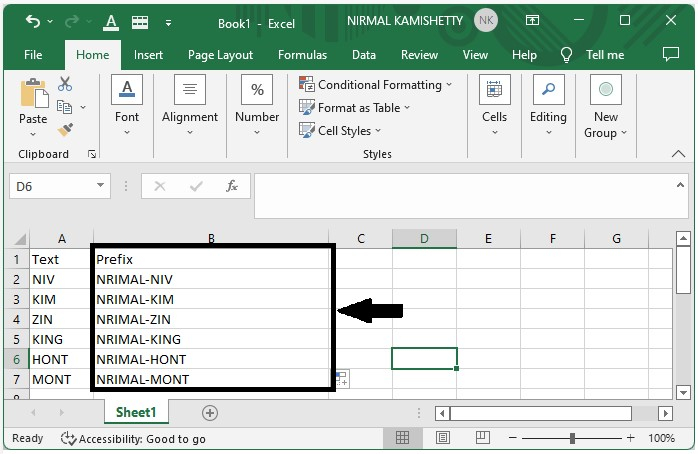## How to Add suffix into Cell Values?

Let see a simple process to add suffix into cell values.

### Step 1:

Let us consider the same data which we used in the above example. To add the prefix, use the formula as Address of cell "message". In our case, the formula will be =A2&"-NIRMAL"

Now click the empty cell where we want to get our result and enter the formula as =A2&"-NIRMAL" and press Enter to get our first result as shown below: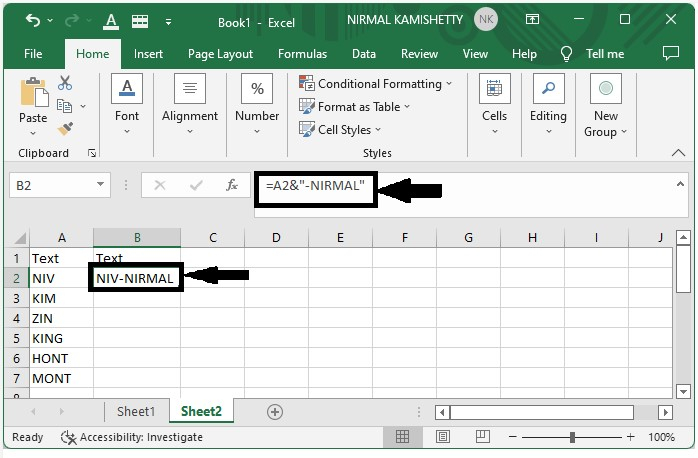### Step 2:

Display all the other results just by dragging down from the corner of the first result till all the selected cells are filled with the new values.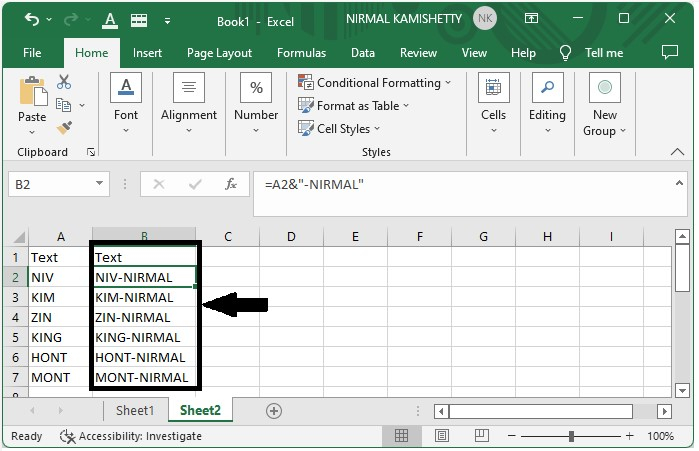## How to Add Both Prefix and Suffix into Cell Values?

Let see a simple process to add both prefix and suffix into cell values

### Step 1:

Let us consider the same data which we used in the above example. To add both prefix and suffix, use the formula as "message" Address of cell "message". In our case, the formula will be ="Mr-"&A2&"-NIRMAL"

Now click the empty cell where you want to get the result and enter the formula as ="Mr-"&A2&"-NIRMAL "and press Enter to get the first result, as shown below: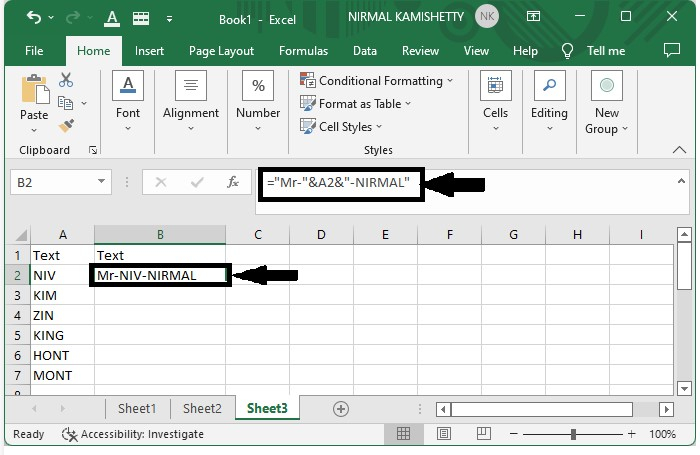### Step 2:

Then, you can display all the other results just by dragging down from the corner of the first result till all the selected cells are filled with the new values. The output will look similar to the one shown below: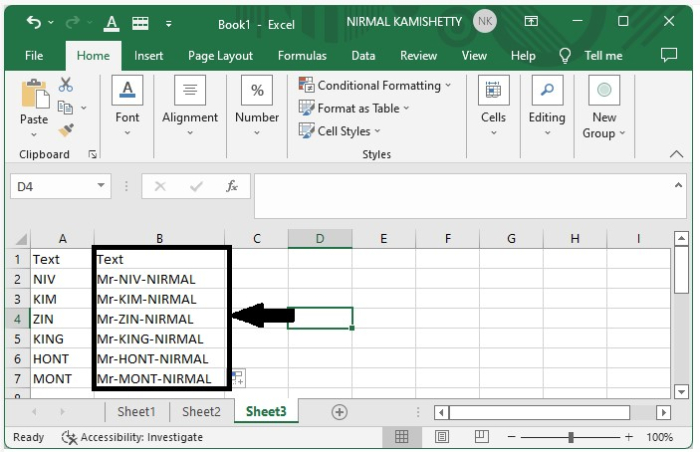This is how you can add prefix and suffix into cell values in a very simple and efficient way.